• 学生以前学过的图形都是多边形(如三角形、长方形、正方形、平行四边形和梯形等)，都是直边图形，像圆这样的曲线图形的面积计算，学生还是第一次接触到，理解起来比较困难。教材直接给出明确的提示，让把圆平均分成...
人教版是从生活情境提出圆面积的概念，体会在实际生活中计算圆面积的必要性。学生以前学过的图形都是多边形(如三角形、长方形、正方形、平行四边形和梯形等)，都是直边图形，像圆这样的曲线图形的面积计算，学生还是第一次接触到，理解起来比较困难。教材直接给出明确的提示，让把圆平均分成若干等份，拼一拼。教师把重点放在了拼和拼后找关系上，忽略了圆的面积的转化过程，求圆的面积人类经过了漫长的过程，其中中国数学家刘徽的“割圆术”以出入相补的归纳思想计算圆的面积，和西方的欧几里得的演绎风格迥然不同却相辅相成。现在的问题是，怎样才能让学生在简单经历割圆术的思想方法的基础上想到如何进行剪拼。我们在人教版、青岛版、北师大版教材上都能看到“割圆术”的影子，怎样在求圆的面积时从这个角度进行教学呢？我们可以尝试这样引导：师：怎样计算圆的面积呢？生：转化成已经学过的图形。师：可圆是曲线图形，不能直接转化成学过的图形，怎么办呢？生：……师：虽然不能直接转化，我们可以用学过的图形进行研究，比如我们在圆内画一个最大的正方形，这个正方形的面积和圆的面积比较？生：圆的面积大于里面正方形的面积。师：这一点我们在圆的认识时就知道了，而且还知道……？生：圆的面积小于外接正方形的面积。师：如果内接和外接的正多边形边越多，我们会发现？课件出示，生：最后和圆重合，就是圆的面积。师：我们试着把圆分割一下，正方形的时候分割成了四个扇形，随着正多边形的边数不断增多，把圆分割成了很多什么图形？生1：小三角形，生2：终于分成了学过的图形。师：正多边形的边数越多，越接近三角形，把这些三角形取下来拼一拼看看你们能发现什么？……事实上，把圆转化成直边图形单靠学生主动探究是很难实现的，从极限的角度他们是很难跨过这个思想鸿沟的，即使按照上述的方法引导有意识的，能朝着正确的方向去做，但最后也会因为数量和表象达不到效果，或者从宏观上解释：是现实操作与理想数学之间的冲突导致的难以持续研究。所以我认为，圆的面积是前面平面图形面积教学的一次全面提升，它应该是让学生经历和掌握圆面积公式的由来，更重要的是体会，平面图形间从“无限”的角度能够实现转化，以及了解有关圆的历史探究知识，在思维上是一次飞跃。(化曲为直、怎么变直的？变直的过程是课堂上研究的重点)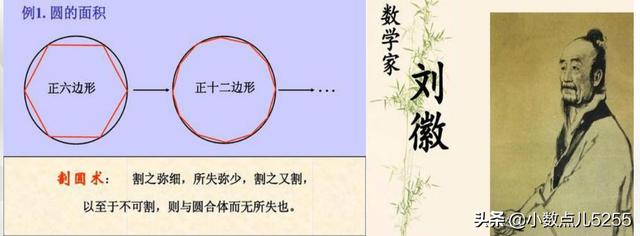最早计算圆面积的是中国数学家刘徽，距离我们近二千年，比祖冲之稍早一些，他写的一本数学著作《九章算术》。刘徽最突出的数学成就就是“割圆术”，祖冲之就是利用刘徽的割圆术发现圆周长与直径的比值是3倍多一些，方便我们计算圆的周长。而刘徽利用割圆术发现圆面积的计算方法，面积的计算公式是刘徽的贡献。他把圆分割为若干小扇形，他从正六边形出发，将边数逐次加倍，并逐步计算正多边形的面积。他说“割之弥细，所失弥少，割之又割，以至于不可割，则与圆周合体而无所失”。圆面积的计算还表现刘徽另一数学原理“出入相补”：一个几何图形被分割为若干后，面积或体积保持不变。此时刘徽已有极限思想。史宁中教授指出，数学最上位的思想主要是归纳和演绎，“几何测量”教学中，教学的主体思想不应该忽视演绎推理，那就是重视让学生经历公式(算法)的形成过程。
展开全文• 微积分是一种非常重要的“数学分析”思想(方法)，在许多领域中都有应用，比如：计算平面面积曲线长度、空间图形的体积、旋转曲面面积和物理学中的“微元法”等。而如何用好“微积分”是这部分学习的重点。要用好微...
微积分是一种非常重要的“数学分析”思想(方法)，在许多领域中都有应用，比如：计算平面面积、曲线长度、空间图形的体积、旋转曲面面积和物理学中的“微元法”等。而如何用好“微积分”是这部分学习的重点。要用好微积分，关键是理解透彻“微分-differential”和“定积分-Integral”的定义。微积分在英文中有时又被称为“Infinitesimal calculus”，即“无穷小量微积分”，这个名字从一定意义上可以帮助我们记忆“微积分”思想：在微观上上研究无穷小量的特征，找出规律，然后回到宏观上计算结果，控制误差。具体方法上，可以参考“Riemann积分”分为五步：分割、取点、近似、求和(求定积分)、分析误差。一、分割分割是微积分方法的第一步，也是微积分应用中非常重要的一步。算法中有“分而治之”的策略(Divide-and-conquer algorithms)，微积分的“分割”也正暗合这种思想。另外所谓“微观化”，通俗理解就是取待研究的对象的一小部分作为单元，放大了仔细研究，找出特征，然后再总结整体规律。而微积分的“分割”也正是这个“取一小部分作为单元”。普遍来说，有两种分割方式：直角坐标系分割和极坐标系分割。1.直角坐标系分割对于直角坐标系分割，我们已经和熟悉了，前面将定积分定义的时候，就是在直角坐标系下用“矩形逼近”的方法来计算曲线与x轴围成的面积。它是沿x轴分割成n小段{Δxi}，即在直角坐标系下分割是按自变量进行分割。当然，直角坐标系下也可以沿y轴分割，本质上，直角坐标系中沿x轴分割和沿y轴分割意义是一样的。将沿y轴分割看作是：将函数关系反转，同时也将坐标轴反转。2，极坐标系分割同样地，极坐标也是按自变量分割。只是，直观上看，与直角坐标系的分割差异较大。如下图：显然，极坐标分割的单元形状类似三角形而不是梯形或矩形。总结：不论是什么坐标系，都是按自变量进行分割。这是由函数的映射关系决定的，已知自变量，通过函数运算，就可以得到函数值。从图形上来看，这样的分割可以使每个分割单元“不规则的边”的数量最小，最好是只有一条不规则的边。选择好了坐标系，分割就不是问题了，所以，在研究实际问题建模的时候，重要的是选取合适的坐标系。二、取点根据积分的定义，取点具有任意性。但是，在实际应用中，为了简化计算或定性分析，我们往往会取一些特殊点，比如左端点或右端点。比如，为了证明这个不等式，我们会把左右两端的式子当作两条曲线的积分，而将中间的和式当作矩形之和，而每个矩形的左右两端点分别落在左右两条曲线上。此外，Darboux Integral也是取得左右两端点。三、近似近似是微积分方法最重要的一步。通过“分割”，有了微观上的“单元”后，这个“单元”还是不太适合直接研究，因为它不规则，只有通过近似，将这个不规则的“单元”近似为一个“规则的单元”，这样才能继续下一步研究。这么说来，“近似”是整个微积分中最有创意，最需要发挥人的联想能力的一步。1，不规则近似为规则可以这么说：近似就是在微观上将不规则的“单元”替换为规则的“单元”。回到面积法，我们无法直接计算一个曲边图形的面积，但是在微观单元上，我们可以用一个相似的直边图形来替代它。直观上看，只要这个微观单元足够小，这个替代的误差也就足够小。也就是说，这个替代某种意义上是可行的，误差是可控制的。前面在讲“分割”的时候说“要使不规则的边的数量尽可能小”，实际上也就是方便做“近似”。2，直线代曲线更具体一点，在前面介绍的定积分定义和上面的“极坐标图形”问题，可以发现这两类问题近似的实际就是“用直线替代曲线”。在直角坐标系中，这么替代后，分割单元由曲边梯形变成了斜边梯形；极坐标系中，这么替代后分割单元由曲边三角形变成了普通三角形。这一步做完后，你会发现在微观上，原来不可计算的问题变成了可计算的问题了。注意，在极坐标系中，计算面积时，既可以用“三角形近似”(Triangle)，也可以用“圆弧近似”(Arc)，后面将讨论这两种近似的误差是一致的。3，套用计算公式之所以要将不规则单元替换为规则单元，是因为规则单元可以套用计算公式。替换完成后，下一步就是针对待求解的问题，对“规则单元”套用已知的公式。待求解的问题不同，套用的公式显然也不同。比如：1)Riemann和定义的例子待求解的是在区间[a,b]上曲线与x轴围成的面积，因此套用的是平面面积公式。2)极坐标系曲线积分(上图)待求解的是在区间[θ1,θ2]上曲线与原点围成的面积，因此套用的是圆弧面积公式。3)平面曲线长度平面曲线在微观上近似为一段段“斜线”(切线)，它遵循的是“直角三角形斜边与直边的公式”，即“Pythagoras定理”：4)极坐标曲线长度注意，不能直接使用弧长公式这个公式的推导过程中用到了π，而π本身就是近似得到的。类似地，我们也可推广到旋转体的体积和表面积。四、求和前面几步都是在微观层面进行的，只有通过“求和”(Riemann和)才能回到宏观层面。其中，Fi表示各个微观单元的公式五、误差分析近似”是发挥人联想能力的时候，但联想完了之后，我们要证明这种“近似”是可行的，即证明“误差在可接受范围内”。当然，对于误差的计算是要回到宏观层面上来的。一是我们原本要研究的就是一个宏观问题，最后的计算结果只有回到宏观上看才有意义；二是微观上的小误差有可能累积到宏观上变成大误差，正所谓“差之毫厘谬以千里”。1，平面曲线积分误差分析在“定积分”那一节，我通过“无穷小的运算”证明了“梯形近似”的误差 ϵ=O(Δx) ，同时也证明了“矩形近似与梯形近似的误差在同一个级别—— O(Δx) ”。2，极坐标曲线积分误差分析现在我们来证明极坐标曲线积分的“三角形近似”和“圆弧近似”的误差 ϵ=O(Δx)。1)圆弧近似(Arc)先用弧线代曲线误差为再算单元面积有单元面积公式可知，Si与Δθi是一次线性关系，即 Si∼Δθi ，那么用弧形面积近似后误差求和后总误差为注意，这里为了计算方便，假设各子区域的误差相等。所以，当Δθ→0时，ϵ→02)三角形近似(Triangle)先用直线代曲线，修改面积公式同样地，可以计算误差为：根据无穷小的替换此外，通过无穷小的替换，也可以证明这两个面积相等(Riemann和)这里关于三角形近似与圆弧近似的论述，可能是因果颠倒的，但是能方便理解。3，曲线长度计算的误差分析1)“Δx”代曲线可行吗？在计算平面曲线的积分时，我们不仅用“Δl”来代替曲线(梯形近似)，而且为了简化计算，直接用“Δx”来代替曲线(矩形近似)。有没有很惊讶，这两种近似在求面积的时候误差是一个级别的(等价无穷小)。那么，是不是什么情况下都可以这么近似呢？答案是，不能！当我们求曲线长度的时候，如果用“Δx”来代替曲线，那么结果很明显是不对的(误差不可接受)如下图，直观上都可以感受到这个误差之大。在计算曲线长度的时候，我们只用“Δl”来代替曲线，并根据“Pythagoras定理”，将“Δl”换算为“Δx”，如下：2)“Δl”代曲线的误差计算每个单元误差为求和后总误差为注意，这里为了计算方便，假设各小段的误差相等。所以，当Δl→0时，ϵ→03)两种近似的比较六、练习求双纽线(lemniscate)围成的平面区域的面积解：先看lemniscate的图形，它是一个对称图形，只需要计算其中的四分之一区域的面积即可。求心形线(heart-line)围成的平面区域的面积解：先看heart-line的图形，它也是一个对称图形，只需要计算其中的二分之一区域的面积即可。需要注意的是，为了美观，这个图与公式并没对应，按题中公式画出的是一个水平偏右的心形。展开全文• 在之前的文章中我们提到过，微积分就是与曲线有关的两种计算方法即 曲线上切线的斜率 曲线围成的面积 当然，这将主题描述的过于简单，...他们知道如何计算三角形、圆和相关图形的面积，但是任何其他图形是一个新问题
在之前的文章中我们提到过，微积分就是与曲线有关的两种计算方法即

曲线上切线的斜率
曲线围成的面积
当然，这将主题描述的过于简单，因为它强调微积分作为几何的工具，没有说它在科学研究中起着不可或缺的作用。然而，它解释了传统微积分可以划分为两个截然不同的部分：微分学(处理切线的斜率)和积分学(处理面积)。

古希腊人对面积问题非常感兴趣。他们知道如何计算三角形、圆和相关图形的面积，但是任何其他图形是一个新问题。阿基米德应用一种叫做逼近的方法来计算一段抛物线的面积以及其他几个几何量。但近2000年来由阿基米德这个天才自己创造出来的计算法其他人都是无法比拟的。然而，由十七世纪中叶几个欧洲思想家(特别是费马和帕斯卡)开始推动阿基米德留下的逼近法。之后的牛顿和莱布尼兹取得了决定性的突破，他们表明，可以用逼近法计算的量，那么很容易通过使用反导来计算。这一重要发现被称为微积分基本定理。它将主题的两部分结合起来，并且无疑是(正如我们以前说过的)整个数学中最重要的一个事实。

下面的几篇文章我将遵循这个路径。计算似乎在我们的工作中是比较突出的部分，但是实际上，牢牢记住基本的思想比计算方法更加重要。
展开全文定积分
• 3.3.2 平面图形的面积 3.3.3 平行截面面积已知的立体体积 3.3.4 曲线的弧长 3.3.5 微分元素法在物理学中的应用 3.4 二重积分的计算及其应用 3.4.1 利用定积分的微分元素法化二重积分为累次积分 3.4.2 二重积分的微分...
• 以绘制y=|lg（6+x^3）|的曲线为例，其方法如下： 在某张空白工作表中，先输入函数自变量：在A列A1格输入"X="，表明这是自变量，再在A列A2及以后格内逐次从小到大输入自变量各个值；实际输入时候，通常...excel
• 5、在多边形的属性中的信息一项中，显示多边形的面积和周长（图4），现在可以方便计算某 一个区的面积了（只是还得需要造一个多边形区来查看它的属性才能得到这些信息，以后 会不会像其他软件一样，直接有一个面积...
• 微积分是一种非常重要的“数学分析”思想（方法），在许多领域中都有应用，比如：计算平面面积曲线长度、空间图形的体积、旋转曲面面积和物理学中的“微元法”等。而如何用好“微积分”是这部分学习的重点。要用...

微积分是一种非常重要的“数学分析”思想（方法），在许多领域中都有应用，比如：计算平面面积、曲线长度、空间图形的体积、旋转曲面面积和物理学中的“微元法”等。而如何用好“微积分”是这部分学习的重点。要用好微积分，关键是理解透彻“微分-differential”和“定积分-Integral”的定义。微积分在英文中有时又被称为“Infinitesimal calculus”，即“无穷小量微积分”，这个名字从一定意义上可以帮助我们记忆“微积分”思想：在微观上上研究无穷小量的特征，找出规律，然后回到宏观上计算结果，控制误差。具体方法上，可以参考“Riemann积分”分为五步：分割、取点、近似、求和（求定积分）、分析误差。
注：关于定积分的几何应用，这两篇文章——AREAS, VOLUMES OF SOLIDS OF REVOLUTION, LENGTH OF A CURVE, AREAS OF SURFACES OF REVOLUTION, WORK, FLUID PRESSURE和Integral Application，讲解的非常精彩，值得多读。

一、分割

分割是微积分方法的第一步，也是微积分应用中非常重要的一步。算法中有“分而治之”的策略（Divide-and-conquer algorithms），微积分的“分割”也正暗合这种思想。另外所谓“微观化”，通俗理解就是取待研究的对象的一小部分作为单元，放大了仔细研究，找出特征，然后再总结整体规律。而微积分的“分割”也正是这个“取一小部分作为单元”。
普遍来说，有两种分割方式：直角坐标系分割和极坐标系分割。
1，直角坐标系分割
对于直角坐标系分割，我们已经和熟悉了，前面将定积分定义的时候，就是在直角坐标系下用“矩形逼近”的方法来计算曲线与x轴围成的面积。它是沿x轴分割成n小段{Δxi}$\{\Delta x_i \}$，即在直角坐标系下分割是按自变量进行分割。
当然，直角坐标系下也可以沿y轴分割，本质上，直角坐标系中沿x轴分割和沿y轴分割意义是一样的。将沿y轴分割看作是：x=f−1(y)$x=f^{-1}(y)$，将函数关系反转，同时也将坐标轴反转。
2，极坐标系分割
同样地，极坐标也是按自变量分割。只是，直观上看，与直角坐标系的分割差异较大。如下图：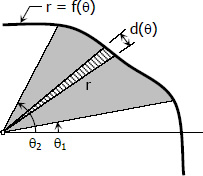显然，极坐标分割的单元形状类似三角形而不是梯形或矩形。
总结：
不论是什么坐标系，都是按自变量进行分割。这是由函数的映射关系决定的，已知自变量，通过函数运算，就可以得到函数值。从图形上来看，这样的分割可以使每个分割单元“不规则的边”的数量最小，最好是只有一条不规则的边。选择好了坐标系，分割就不是问题了，所以，在研究实际问题建模的时候，重要的是选取合适的坐标系。

二、取点

根据积分的定义，取点具有任意性。但是，在实际应用中，为了简化计算或定性分析，我们往往会取一些特殊点，比如左端点或右端点。比如，为了证明这个不等式，我们会把左右两端的式子当作两条曲线的积分，而将中间的和式当作矩形之和，而每个矩形的左右两端点分别落在左右两条曲线上。
∫n+11nn2+x2dx≤∑k=1nnn2+k2≤f(1)+∫n1nn2+x2dx\int_{1}^{n+1}\frac{n}{n^{2}+x^{2}}dx\;\leq \sum_{k=1}^{n}\frac{n}{n^{2}+k^{2}}\;\leq  f(1)+\int_{1}^{n}\frac{n}{n^{2}+x^{2}}dx
此外，Darboux Integral也是取得左右两端点。

三、近似

近似是微积分方法最重要的一步。通过“分割”，有了微观上的“单元”后，这个“单元”还是不太适合直接研究，因为它不规则，只有通过近似，将这个不规则的“单元”近似为一个“规则的单元”，这样才能继续下一步研究。这么说来，“近似”是整个微积分中最有创意，最需要发挥人的联想能力的一步。
1，不规则近似为规则
可以这么说：近似就是在微观上将不规则的“单元”替换为规则的“单元”。回到面积法，我们无法直接计算一个曲边图形的面积，但是在微观单元上，我们可以用一个相似的直边图形来替代它。直观上看，只要这个微观单元足够小，这个替代的误差也就足够小。也就是说，这个替代某种意义上是可行的，误差是可控制的。前面在讲“分割”的时候说“要使不规则的边的数量尽可能小”，实际上也就是方便做“近似”。
2，直线代曲线
更具体一点，在前面介绍的定积分定义和上面的“极坐标图形”问题，可以发现这两类问题近似的实际就是“用直线替代曲线”。在直角坐标系中，这么替代后，分割单元由曲边梯形变成了斜边梯形；极坐标系中，这么替代后分割单元由曲边三角形变成了普通三角形。这一步做完后，你会发现在微观上，原来不可计算的问题变成了可计算的问题了。
注意，在极坐标系中，计算面积时，既可以用“三角形近似”（Triangle），也可以用“圆弧近似”（Arc），后面将讨论这两种近似的误差是一致的。
3，套用计算公式
之所以要将不规则单元替换为规则单元，是因为规则单元可以套用计算公式。
替换完成后，下一步就是针对待求解的问题，对“规则单元”套用已知的公式。待求解的问题不同，套用的公式显然也不同。比如：
1）Riemann和定义的例子
待求解的是在区间[a,b]上曲线与x轴围成的面积，因此套用的是平面面积公式。
Si=hi∗wi=f(ξi)∗Δxi S_i = h_i * w_i = f(\xi_i) * \Delta x_i
2）极坐标系曲线积分（上图）
待求解的是在区间[θ1,θ2]$[\theta_1, \theta_2]$上曲线与原点围成的面积，因此套用的是圆弧面积公式。
Si=12∗r2i∗Δθi=12∗[f(ξi)]2∗Δθi S_i = \frac {1}{2} * r_i^2 * \Delta \theta_i = \frac {1}{2} * [f(\xi_i)]^2 * \Delta \theta_i
3）平面曲线长度
平面曲线在微观上近似为一段段“斜线”（切线），它遵循的是“直角三角形斜边与直边的公式”，即“Pythagoras定理”：
Δli=(Δxi)2+(Δyi)2−−−−−−−−−−−−−√=1+(ΔyiΔxi)2−−−−−−−−−√Δxi\Delta l_i = \sqrt {(\Delta x_i)^2 + (\Delta y_i)^2} = \sqrt{1 + (\frac{\Delta y_i}{\Delta x_i})^2}\; \Delta x_i
4）极坐标曲线长度
dl=(dx)2+(dy)2−−−−−−−−−−−√=d2[r(θ)∗cos(θ)]dθ2+d2[r(θ)∗sin(θ)]dθ2−−−−−−−−−−−−−−−−−−−−−−−−−−−−√dθ=r2(θ)+r′2(θ)−−−−−−−−−−√dθ\mathrm{d}l = \sqrt {(\mathrm{d} x)^2 + (\mathrm{d} y)^2} = \sqrt{\frac{\mathrm{d}^2[r(\theta)*cos(\theta)] }{\mathrm{d} \theta ^ 2} + \frac{\mathrm{d} ^2 [r(\theta)*sin(\theta)]}{\mathrm{d} \theta ^2}}  \; \mathrm{d} \theta\\
= \sqrt{r^2(\theta)+r'^2(\theta)}\; \mathrm{d} \theta
注意，不能直接使用弧长公式Δli=ri∗Δθi=f(ξi)∗Δθi$\Delta l_i = r_i * \Delta \theta_i = f(\xi_i) * \Delta \theta_i$，这个公式的推导过程中用到了π$\pi$，而π$\pi$本身就是近似得到的。
类似地，我们也可推广到旋转体的体积和表面积。

四、求和

前面几步都是在微观层面进行的，只有通过“求和”（Riemann和）才能回到宏观层面。
limλ→0+∑i=0nFi\lim_{\lambda \rightarrow 0^{+}}\sum_{i=0}^{n} F_i
其中，Fi$F_i$表示各个微观单元的公式

五、误差分析

“近似”是发挥人联想能力的时候，但联想完了之后，我们要证明这种“近似”是可行的，即证明“误差在可接受范围内”。当然，对于误差的计算是要回到宏观层面上来的。一是我们原本要研究的就是一个宏观问题，最后的计算结果只有回到宏观上看才有意义；二是微观上的小误差有可能累积到宏观上变成大误差，正所谓“差之毫厘谬以千里”。
1，平面曲线积分误差分析
在“定积分”那一节，我通过“无穷小的运算”证明了“梯形近似”的误差 ϵ=O(Δx)$\epsilon=O(\Delta x)$ ，同时也证明了“矩形近似与梯形近似的误差在同一个级别——  O(Δx)$O(\Delta x)$ ”。传送门-Integral
2，极坐标曲线积分误差分析
现在我们来证明极坐标曲线积分的“三角形近似”和“圆弧近似”的误差  ϵ=O(Δx)$\epsilon=O(\Delta x)$。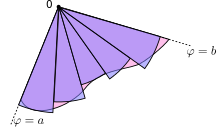1）圆弧近似（Arc）
先用弧线代曲线
Δli=Δri∗Δθi+O[(Δθi)2]=f(ξi)∗Δθi+O[(Δθi)2]\Delta l_i = \Delta r_i * \Delta \theta_i + O[(\Delta \theta_i)^2]= f(\xi_i) * \Delta \theta_i + O[(\Delta \theta_i)^2]
误差为 ϵl=O[(Δθi)2]$\epsilon_l = O[(\Delta \theta_i)^2]$
再算单元面积
Si=12∗r2i∗Δθi=12∗[f(ξi)]2∗Δθi S_i = \frac {1}{2} * r_i^2 * \Delta \theta_i = \frac {1}{2} * [f(\xi_i)]^2 * \Delta \theta_i
有单元面积公式可知，Si$S_i$与Δθi$\Delta \theta_i$是一次线性关系，即 Si∼Δθi$S_i \sim \Delta \theta_i$ ，那么用弧形面积近似后误差ϵi=O[(Δθi)2]$\epsilon_i = O[(\Delta \theta_i)^2]$
求和后总误差为
ϵ=n∗O[(Δθ)2]=1Δθ∗O[(Δθ)2]=O(Δθ)\epsilon = n *O[(\Delta \theta)^2] = \frac {1}{\Delta \theta} * O[(\Delta \theta)^2] = O(\Delta \theta)
注意，这里为了计算方便，假设各子区域的误差相等。
所以，当Δθ→0$\Delta \theta \rightarrow 0$时，ϵ→0$\epsilon \rightarrow 0$
2）三角形近似（Triangle）
先用直线代曲线，修改面积公式
Si=12∗r2i∗sin(Δθi)=12∗[f(ξi)]2∗sin(Δθi) S_i = \frac {1}{2} * r_i^2 * sin(\Delta \theta_i) = \frac {1}{2} * [f(\xi_i)]^2 * sin(\Delta \theta_i)
同样地，可以计算误差为：
ϵi=O[sin2(Δθi)]ϵ=n∗O[sin2(Δθ)]=1Δθ∗O[sin2(Δθ)]\epsilon_i = O[sin^2(\Delta \theta_i)] \\ \epsilon = n *O[sin^2(\Delta \theta)] = \frac {1}{\Delta \theta} * O[sin^2(\Delta \theta)]
根据无穷小的替换
limΔθ→0+sin(Δθ)Δθ=1ϵ=1Δθ∗O[sin2(Δθ)]=O(Δθ)\lim_{\Delta \theta \rightarrow 0^{+}}\frac{sin(\Delta \theta)}{\Delta \theta} = 1 \\
\epsilon = \frac {1}{\Delta \theta} * O[sin^2(\Delta \theta)] = O(\Delta \theta)
此外，通过无穷小的替换，也可以证明这两个面积相等（Riemann和）
StSa=limΔθ→0+∑ni=012∗[f(ξi)]2∗sin(Δθi)limΔθ→0+∑ni=012∗[f(ξi)]2∗Δθi=1 \frac {S_t}{S_a} = \frac {\lim_{\Delta \theta \rightarrow 0^{+}}\sum_{i=0}^{n} \frac {1}{2} * [f(\xi_i)]^2 * sin(\Delta \theta_i)} {\lim_{\Delta \theta \rightarrow 0^{+}}\sum_{i=0}^{n} \frac {1}{2} * [f(\xi_i)]^2 * \Delta \theta_i} = 1
这里关于三角形近似与圆弧近似的论述，可能是因果颠倒的，但是能方便理解。事实上，在该课程第一章“极限论”中，介绍“割圆术”的时候，扈老师就用极限的方法演示了三角形近似与圆弧近似在极限情况下是相等的，有兴趣的可以再去观看那一段视频。
3，曲线长度计算的误差分析
1）“Δx$\Delta x$”代曲线可行吗？
在计算平面曲线的积分时，我们不仅用“Δl$\Delta l$”来代替曲线（梯形近似），而且为了简化计算，直接用“Δx$\Delta x$”来代替曲线（矩形近似）。有没有很惊讶，这两种近似在求面积的时候误差是一个级别的（等价无穷小）。那么，是不是什么情况下都可以这么近似呢？
答案是，不能！
当我们求曲线长度的时候，如果用“Δx$\Delta x$”来代替曲线，那么结果很明显是不对的（误差不可接受）
l=∫x2x0dx=x2−x0l = \int_{x_0}^{x_2}\mathrm{d} x=x_2 - x_0
如下图，直观上都可以感受到这个误差之大。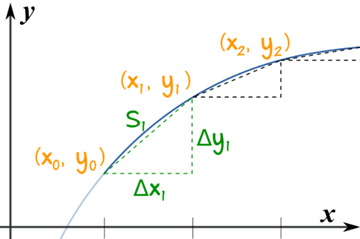在计算曲线长度的时候，我们只用“Δl$\Delta l$”来代替曲线，并根据“Pythagoras定理”，将“Δl$\Delta l$”换算为“Δx$\Delta x$”，如下：
l=∫badl=∫x2x01+(dydx)2−−−−−−−−√dx=∫y2y01+(dxdy)2−−−−−−−−√dyl = \int_{a}^{b}\mathrm{d} l=\int_{x_0}^{x_2}\sqrt{1 + (\frac{\mathrm{d} y}{\mathrm{d} x})^2}\; \mathrm{d} x=\int_{y_0}^{y_2}\sqrt{1 + (\frac{\mathrm{d} x}{\mathrm{d} y})^2}\; \mathrm{d} y
2）“Δl$\Delta l$”代曲线的误差计算
每个单元误差为 ϵi=O[(Δli)2]$\epsilon_i = O[(\Delta l_i)^2]$
求和后总误差为
ϵ=n∗O[(Δl)2]=1Δl∗O[(Δl)2]=O(Δl)\epsilon = n *O[(\Delta l)^2] = \frac {1}{\Delta l} * O[(\Delta l)^2] = O(\Delta l)
注意，这里为了计算方便，假设各小段的误差相等。
所以，当Δl→0$\Delta l \rightarrow 0$时，ϵ→0$\epsilon \rightarrow 0$
3）两种近似的比较
Δli=(Δxi)2+(Δyi)2−−−−−−−−−−−−−√=1+[f′(ξi)]2−−−−−−−−−−√Δxi\Delta l_i = \sqrt {(\Delta x_i)^2 + (\Delta y_i)^2} = \sqrt{1 + [f'(\xi_i)]^2}\; \Delta x_i
limλ→0+∑ni=01+[f′(ξi)]2−−−−−−−−−−√Δxilimλ→0+∑ni=0Δxi≠1 \frac {\lim_{\lambda \rightarrow 0^{+}}\sum_{i=0}^{n} \sqrt{1 + [f'(\xi_i)]^2}\; \Delta x_i} {\lim_{\lambda \rightarrow 0^{+}}\sum_{i=0}^{n} \Delta x_i} \ne 1

六、课后练习

Exercise 7-5-1
求双纽线（lemniscate）ρ2=2a2cos(2θ)$\rho^2 = 2a^2cos(2\theta)$围成的平面区域的面积
解：先看lemniscate的图形，它是一个对称图形，只需要计算其中的四分之一区域的面积即可。

%matplotlib inline
import numpy as np
import matplotlib.pyplot as plt
alpha = 1
theta = np.linspace(0, 2*np.pi, num=1000)
x = alpha * np.sqrt(2) * np.cos(theta) / (np.sin(theta)**2 + 1)
y = alpha * np.sqrt(2) * np.cos(theta) * np.sin(theta) / (np.sin(theta)**2 + 1)
plt.plot(x, y)
plt.grid()
plt.show()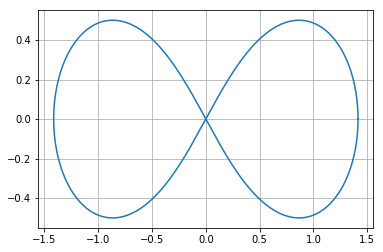#Exercise 7-5-1
from sympy import *
init_printing()
t, a = symbols('t a')
f = a ** 2 * cos(2 * t)
4 * integrate(f, (t, 0, pi / 4))

2a22 a^{2}

Exercise 7-5-2
求心形线（heart-line）ρ=a∗(1+cos(θ))$\rho = a * (1 +cos(\theta))$围成的平面区域的面积
解：先看heart-line的图形，它也是一个对称图形，只需要计算其中的二分之一区域的面积即可。需要注意的是，为了美观，这个图与公式并没对应，按题中公式画出的是一个水平偏右的心形。

import matplotlib.pyplot as plt
import numpy as np
t = np.arange(0,2*np.pi, 0.1)
x = 16*np.sin(t)**3
y = 13*np.cos(t)-5*np.cos(2*t)-2*np.cos(3*t)-np.cos(4*t)
plt.plot(x,y)
plt.grid()
plt.show()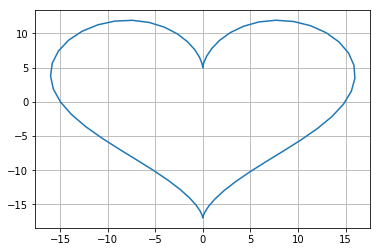#Exercise 7-5-2
from sympy import *
init_printing()
t, a = symbols('t a')
rho = a * (1 + cos(t))
integrate(1 / 2 * rho ** 2, (t, 0, pi))

0.75πa20.75 \pi a^{2}

#Exercise 7-5-3
from sympy import *
init_printing()
x = Symbol('x')
f = x ** 2
g = sqrt(x)
a, b = solve(Eq(f, g), x)
a, b, integrate(f - g, (x, a, b))

#Exercise 7-5-4
%matplotlib inline
import numpy as np
import matplotlib.pyplot as plt
a = 1
t = np.linspace(0, 2 * np.pi)
x = a * (t - np.sin(t))
y = a * (1 - np.cos(t))
plt.plot(x, y)
plt.grid()
plt.show()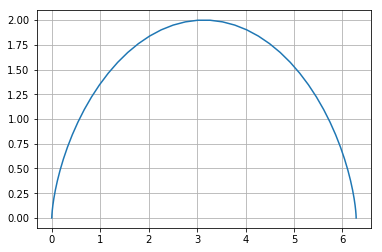Exercise 7-5-4
计算旋轮线的弧长
解：本题看似简单，实际上直接用sympy是计算不出来的。
根据弧长积分公式dl=1+(dydx)2−−−−−−−−√dx$\mathrm{d}l = \sqrt{1 + (\frac{\mathrm{d} y}{\mathrm{d} x})^2}\; \mathrm{d}x$
将积分元替换为t得：
l=a∫2π01−cos(t)−−−−−−−−√dt=2a∫π01−cos(t)−−−−−−−−√dtl = a \int_{0}^{2\pi }\sqrt{1-cos(t)} \; dt = 2a \int_{0}^{\pi }\sqrt{1-cos(t)} \; dt
再次利用三角函数关系，进行有理化
l=2a∫π2−π21−sin(π2+θ)−−−−−−−−−−−−√dθ=2a∫π2−π21−2sin(π4+θ2)cos(π4+θ2)−−−−−−−−−−−−−−−−−−−−−−−√dθ=2a∫π2−π2|sin(π4+θ2)−cos(π4+θ2)|dθl= 2a \int_{-\frac{\pi}{2}}^{\frac{\pi}{2} }\sqrt{1-sin(\frac{\pi}{2}+\theta )} \; d\theta \\
= 2a \int_{-\frac{\pi}{2}}^{\frac{\pi}{2} }\sqrt{1-2sin(\frac{\pi}{4}+\frac{\theta}{2} )cos(\frac{\pi}{4}+\frac{\theta}{2} )} \; d\theta  \\
= 2a \int_{-\frac{\pi}{2}}^{\frac{\pi}{2} }|sin(\frac{\pi}{4}+\frac{\theta}{2} )-cos(\frac{\pi}{4}+\frac{\theta}{2} )| \; d\theta

#Exercise 7-5-5
from sympy import *
init_printing()
t, a = symbols('t a')
r = a * (1 + cos(t))
f = sqrt(r ** 2 + diff(r, t) ** 2)
f

a2(cos(t)+1)2+a2sin2(t)−−−−−−−−−−−−−−−−−−−−−√\sqrt{a^{2} \left(\cos{\left (t \right )} + 1\right)^{2} + a^{2} \sin^{2}{\left (t \right )}}

注意，这个f不能直接用sympy积分，与上题类似进行化简，即可得到积分结果为8a。

#Exercise 7-5-6
from sympy import *
init_printing()
t, a, c = symbols('t a c')
x = a * cos(t)
y = a * sin(t)
z = c * t
f = sqrt(x.diff(t) ** 2 + y.diff(t) ** 2 + z.diff(t) ** 2)
integrate(f, (t, 0, 2 * pi))

2πa2+c2−−−−−−√2 \pi \sqrt{a^{2} + c^{2}}

#Exercise 7-5-9
x = Symbol('x')
f = pi * sin(x) ** 2
integrate(f, (x, 0, pi))

π22\frac{\pi^{2}}{2}

#Exercise 7-5-10
x = Symbol('x')
y = x ** 3 / 3
perimeter = 2 * pi * y
Dlx = sqrt(1 + diff(y, x) ** 2)
f = perimeter * Dlx
integrate(f, (x, 0, 1))

−π9+2π92√- \frac{\pi}{9} + \frac{2 \pi}{9} \sqrt{2}

Exercise 7-5-11
求平面曲线x2a2+y2b2=1$\frac {x^2}{a^2} + \frac {y^2}{b^2} = 1$围成的区域的面积
解：它实际是一个椭圆，a和b分别表示长短轴。将它转为参数方程，求解更容易

#Exercise 7-5-11
from sympy import *
init_printing()
t, a, b = symbols('t a b')
x = a * cos(t)
y = b * sin(t)
f = y * diff(x, t)
integrate(f, (t, 0, 2 * pi))

−πab- \pi a b

#Exercise 7-5-12
from sympy import *
init_printing()
x = Symbol('x')
y = 1 - x ** 2
a, b = solve(y, x)
f = pi * y ** 2
integrate(f, (x, a, b))

16π15\frac{16 \pi}{15}

#Exercise 7-5-13
from sympy import *
init_printing()
x = Symbol('x')
y = sin(x)
f = pi * y ** 2
integrate(f, (x, 0, pi))

π22\frac{\pi^{2}}{2}

#Exercise 7-5-14
from sympy import *
init_printing()
y = Symbol('y')
eq = Eq(y ** 2 / 2, y + 4)
a, b = solve(eq)
f = y ** 2 / 2 - (y + 4)
a, b, integrate(f, (y, a, b))

%matplotlib inline
import numpy as np
import matplotlib.pyplot as plt
y = np.linspace(-3, 5)
x1 = y ** 2 / 2
x2 = y + 4
plt.plot(x1, y)
plt.plot(x2, y)
plt.grid()
plt.show()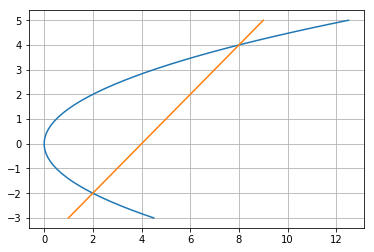展开全文python
• 其实《几何画板》中提供了通过键盘命令（几个标点符号键）直接输入几何图形的方法。 句号( 。 ) —— 绘制点 逗号( ， ) —— 绘制圆 斜杠( / ) —— 绘制线（包括线段、射线和直线，它们各类型之间可通过重复点击来...
• 23.4 函数曲线下方的面积 228 23.5 函数的斜率 229 第24章 定义匿名函数 234 24.1 lambda表达式的语法 234 24.2 lambda表达式的辖域和语义 235 24.3 lambda表达式的语用 237 第五部分 生成递归 第25章 一种新的递归...
• Jalpha查找表 14.3.3示例——将数据映射到颜色或透明度 14.4选择一个...计算可视化工具 16.1剖面图 16.1.1slice函数 16.1.2切片等值线图 16.1.3切片流线图 16.2表现流动特征 16.2.1流线图 ...MAPGIS
• 5、在多边形的属性中的信息一项中，显示多边形的面积和周长（图4），现在可以方便计算某 一个区的面积了（只是还得需要造一个多边形区来查看它的属性才能得到这些信息，以后 会不会像其他软件一样，直接有一个面积...
• ## surfer 9.11 汉化.part1

热门讨论 2011-03-23 08:46:07
5、在多边形的属性中的信息一项中，显示多边形的面积和周长（图4），现在可以方便计算某 一个区的面积了（只是还得需要造一个多边形区来查看它的属性才能得到这些信息，以后 会不会像其他软件一样，直接有一个面积...
• ## surfer9.11汉化.part3

热门讨论 2011-03-23 08:47:20
5、在多边形的属性中的信息一项中，显示多边形的面积和周长（图4），现在可以方便计算某 一个区的面积了（只是还得需要造一个多边形区来查看它的属性才能得到这些信息，以后 会不会像其他软件一样，直接有一个面积...
• 实例116　自定义抽象类计算圆形的面积 实例117　利用接口实现选择不同的语言 实例118　使用密封类密封用户信息 实例119　通过重写虚方法实现加法运算 实例120　通过类的多态性确定人类的说话行为 5.4　迭代器和分部...C#
• 实例116　自定义抽象类计算圆形的面积 实例117　利用接口实现选择不同的语言 实例118　使用密封类密封用户信息 实例119　通过重写虚方法实现加法运算 实例120　通过类的多态性确定人类的说话行为 5.4　迭代器和分部...C#
• 实例116　自定义抽象类计算圆形的面积 实例117　利用接口实现选择不同的语言 实例118　使用密封类密封用户信息 实例119　通过重写虚方法实现加法运算 实例120　通过类的多态性确定人类的说话行为 5.4　迭代器和分部...C#
• 　实例116 自定义抽象类计算圆形的面积 141 　实例117 利用接口实现选择不同的语言 143 　实例118 使用密封类密封用户信息 145 　实例119 通过重写虚方法实现加法运算 146 　实例120 通过类的多态性确定人类的....net c#
• 实例116 自定义抽象类计算圆形的面积 141 实例117 利用接口实现选择不同的语言 143 实例118 使用密封类密封用户信息 145 实例119 通过重写虚方法实现加法运算 146 实例120 通过类的多态性确定人类的说话 行为 147 ...
• 　无论要设计网络、商业规划图还是办公室建筑图，Visio 2007都能够把你思想转换成结构精妙图表和绘图：这本内容全面参考书向读者显示了它是如何做到这些：通过本书，读者将学习如何在lT、建筑、工程和商业...
• 　无论要设计网络、商业规划图还是办公室建筑图，Visio 2007都能够把你思想转换成结构精妙图表和绘图：这本内容全面参考书向读者显示了它是如何做到这些：通过本书，读者将学习如何在lT、建筑、工程和商业...
• 　无论要设计网络、商业规划图还是办公室建筑图，Visio 2007都能够把你思想转换成结构精妙图表和绘图：这本内容全面参考书向读者显示了它是如何做到这些：通过本书，读者将学习如何在lT、建筑、工程和商业...
• 　无论要设计网络、商业规划图还是办公室建筑图，Visio 2007都能够把你思想转换成结构精妙图表和绘图：这本内容全面参考书向读者显示了它是如何做到这些：通过本书，读者将学习如何在lT、建筑、工程和商业...
• 　无论要设计网络、商业规划图还是办公室建筑图，Visio 2007都能够把你思想转换成结构精妙图表和绘图：这本内容全面参考书向读者显示了它是如何做到这些：通过本书，读者将学习如何在lT、建筑、工程和商业...